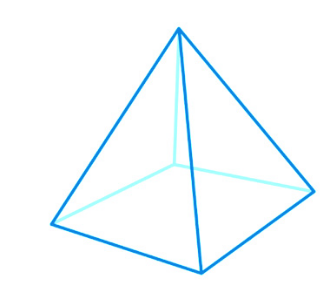# Surface Area of a Square Pyramid Formula

Square pyramid is also called as a polyhedron with a square base and surrounded by four triangular faces.The apex is the point where all the four faces meet. The faces of the pyramid attaches the based with the apex. The sum of the surface area of the base as well as the four triangular face is the surface area of the square pyramid formula.

This is how a rectangular pyramid looks like:The formula for the same is given as:

$\large Surface\;area\;of\;a\;square\;pyramid= 2bs+b^{2}$

### Solved example

Question: What is the surface area of the square pyramid which has the base length of 8 cm and side 5 cm?

Solution:

Given,
Base length = 8 cm
Side length = 5 cm

Using the formula:

$$\begin{array}{l}Surface\;area\;of\;a\;square\;pyramid= 2bs+b^{2}\end{array}$$
$$\begin{array}{l}=2\times 8\times 5+64=144\;cm^{2}\end{array}$$